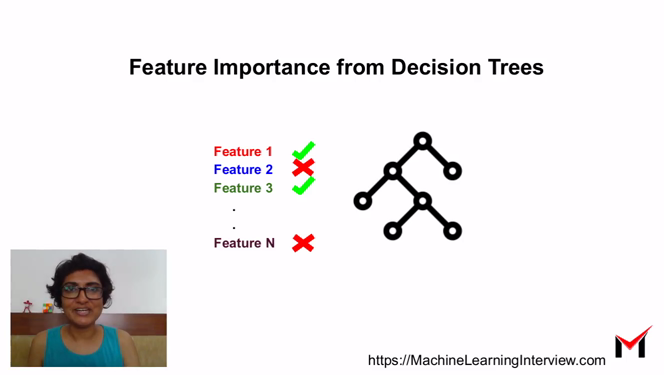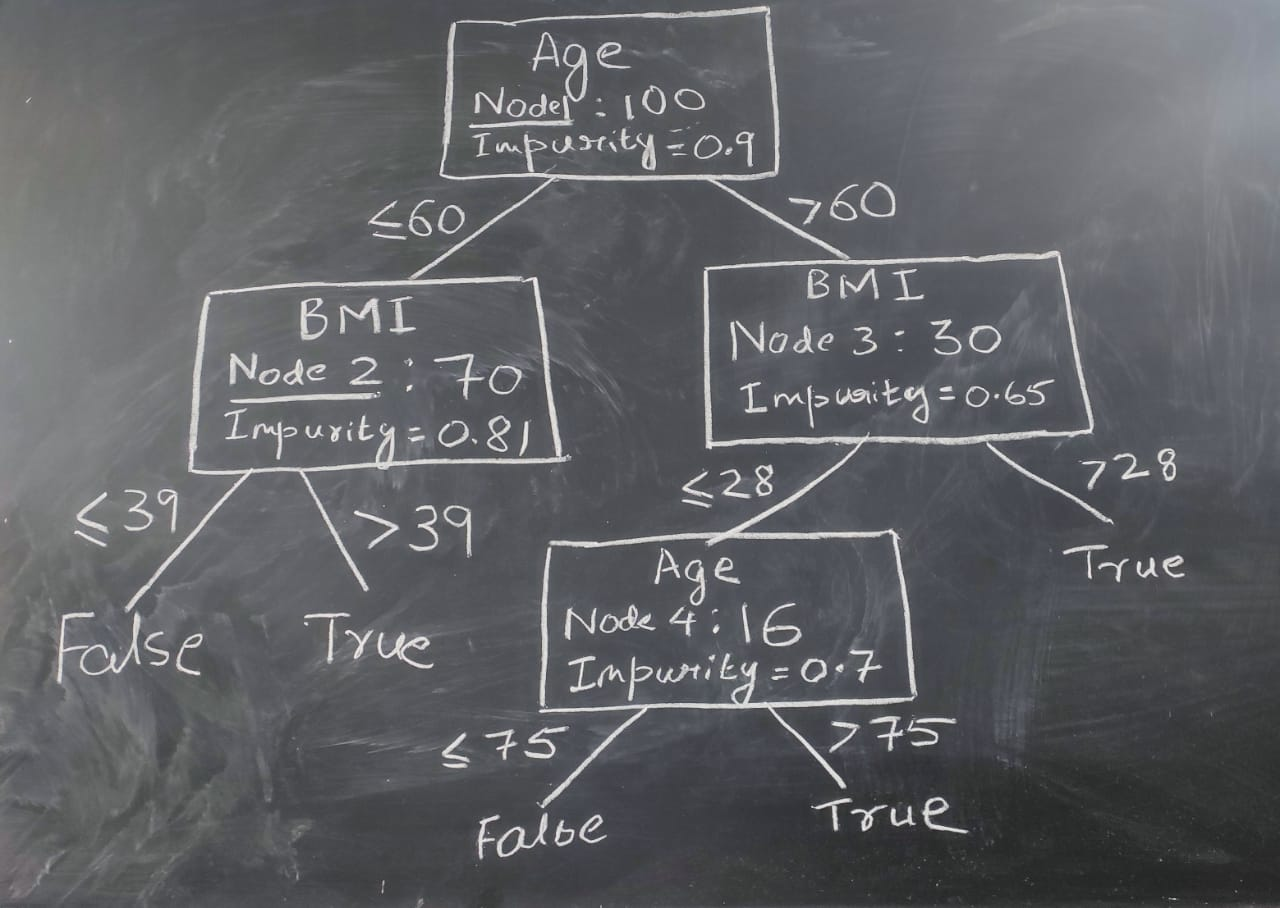# Learning Feature Importance from Decision Trees and Random Forests

This video shows the process of feature selection with Decision Trees and Random Forests.##### Why do we need Feature Selection?

Often we end up with large datasets with redundant features that need to be cleaned up before making sense of the data.  Check out this related article on Recursive Feature Elimination that describes the challenges due to redundant features.

##### How does the Decision Tree Learning Algorithm work?

The decision tree algorithms works by recursively partitioning the data until all the leaf partitions are homegeneous enough.

There are different measures of homogenity or Impurity that measure how pure a node is. A node where all instances have the same label is fully pure, while a node with mixed instances of different labels is impure. Some popular impurity measures that measure the level of purity in a node are:

• Gini Index
• Information Gain
• Variance reduction

The learning algorithm itself can be summarized as follows:

```Until each leaf node is homegeneous enough:

Find the best split for the leaf impure node```
##### How do we Compute feature importance from decision trees?

The basic idea for computing the feature importance for a specific feature involves computing the impurity metric of the node subtracting the impurity metric of any child nodes. This gives us a measure of the reduction in impurity due to partitioning on the particular feature for the node.

```FI for a feature for a specific Node=

Node_impurity * N_node / N_total

–  Impurity_left * N_right / N_total

–  impurity_right * N_left / N_total```

Lets look at an Example: Consider the following decision tree:Let’s say we want to construct a decision tree for predicting from patient attributes such as Age, BMI and height, if there is a chance of hospitalization during the pandemic.

FI(Age)= FI Age from node1 + FI Age from node4

FI(BMI)= FI BMI from node2 + FI BMI from node3

FI(Height)=0

Further, it is customary to normalize the feature importance:

FI(Total)= FI(Age)+ FI(BMI) + FI(Height)

FI_norm(Age)=FI(Age)/FI(Total)

FI_norm(BMI)=FI(BMI)/FI(Tot)

FI_norm(Height)=FI(H)/FI(Total)

##### How do we Compute feature importance from Random Forests?

Recall that building a random forests involves building multiple decision trees from a subset of features and datapoints and aggregating their prediction to give the final prediction.

The feature importance in the case of a random forest can similarly be aggregated from the feature importance values of individual decision trees through averaging.

##### Code references for python implementation

Scikitlearn decision tree classifier has an output attribute `feature_importances_` that can be readily used to get the feature importance values from a trained decision tree model.